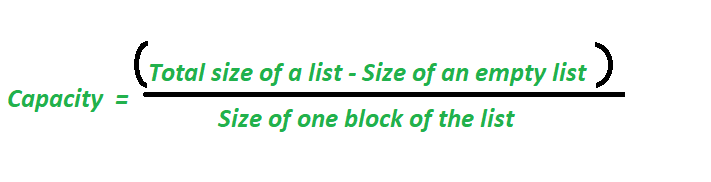# How to find the current capacity of a list in Python

• Last Updated : 01 May, 2020

List in Python is mainly implementation of dynamic sized arrays (like ArrayList in Java or vector in C++). Capacity of a list means number of elements a list can store at a specific time. When we append an element to a list, it will store the element if there is size is less than capacity. If current capacity exceeds, then lists resize and allocate extra space for future insertions (Please see how lists work in Python for details)
The formula for finding the capacity of a list and space left in the list:Here,
size of an empty list means how many bits are allocated to an empty list and it varies system to system .
size of one block of the list also varies from system to system.

Length of the list means the number of blocks of space which is filled

Example 1:

 `# program to find capacity of the list`` ` `import` `sys``l ``=` `[]``size ``=` `sys.getsizeof(l)``print``(``"size of an empty list :"``, size)`` ` `# append an element in the list``l.append(``1``)`` ` `# calculate total size of the list after appending one element``print``(``"Now total size of a list :"``, sys.getsizeof(l))`` ` `# calculate capacity of the list after appending one element``# Considering block size as 8.``capacity ``=` `(sys.getsizeof(l)``-``size)``/``/``8``print``(``"capacity of the list is:"``, capacity)``print``(``"length of the list is :"``, ``len``(l))`` ` `# calculate space left the list after appending one element``spaceleft ``=` `((sys.getsizeof(l)``-``size)``-``len``(l)``*``8``)``/``/``8``print``(``"space left in the list is:"``, spaceleft)`

OUTPUT

```size of an empty list : 64
Now total size of a list : 96
capacity of the list is: 4
length of the list is: 1
space left in the list is: 3```

Example 2:

 `# program to find capacity of the list``import` `sys``l ``=` `[]``size ``=` `sys.getsizeof(l)``print``(``"size of an empty list :"``, size)`` ` `# append four element in the list``l.append(``1``)``l.append(``2``)``l.append(``3``)``l.append(``4``)`` ` `# calculate total size of the list after appending four element``print``(``"Now total size of a list :"``, sys.getsizeof(l))`` ` `# calculate capacity of the list after appending four element``c ``=` `(sys.getsizeof(l)``-``size)``/``/``8``print``(``"capacity of the list is:"``, c)``print``(``"length of the list is:"``, ``len``(l))`` ` `# calculate space left the list after appending four element``spaceleft ``=``((sys.getsizeof(l)``-``size)``-``len``(l)``*``8``)``/``/``8``print``(``"space left in the list is:"``, spaceleft)`

OUTPUT

```size of an empty list : 64
Now total size of a list : 96
capacity of the list is: 4
length of the list is: 4
space left in the list is: 0
```

Example 3:

 `# program to find capacity of the list``import` `sys``l ``=` `[]``size ``=` `sys.getsizeof(l)``print``(``"size of an empty list :"``, size)`` ` `# append five element in the list``l.append(``1``)``l.append(``2``)``l.append(``3``)``l.append(``4``)``l.append(``5``)`` ` `# calculate total size of the list after appending five element``print``(``"Now total size of a list :"``, sys.getsizeof(l))`` ` `# calculate capacity of the list after appending five element``c ``=` `(sys.getsizeof(l)``-``size)``/``/``8``print``(``"capacity of the list is:"``, c)``print``(``"length of the list is:"``, ``len``(l))`` ` `# calculate space left the list after appending five element``spaceleft ``=``((sys.getsizeof(l)``-``size)``-``len``(l)``*``8``)``/``/``8``print``(``"space left in the list is:"``, spaceleft)`

OUTPUT

```size of an empty list : 64
Now total size of a list : 128
capacity of the list is: 8
length of the list is: 5
space left in the list is: 3
```

My Personal Notes arrow_drop_up